# 数据结构——堆结构

2019-01-05

## 1 堆结构

（英语：Heap）是计算机科学中一类特殊的数据结构的统称。堆通常是一个可以被看做一棵树的数组对象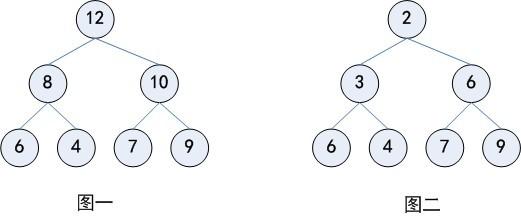1. 堆中任意节点的值总是不大于(不小于)其子节点的值；
2. 堆总是一棵完全树，即除了最底层，其他层的节点都被元素填满，且最底层尽可能地从左到右填入。

## 2 二叉堆

• 当父节点的键值总是大于或等于任何一个子节点的键值时为最大堆
• 当父节点的键值总是小于或等于任何一个子节点的键值时为最小堆

• 索引为i的左孩子的索引是 (2*i+1);
• 索引为i的右孩子的索引是 (2*i+2);
• 索引为i的父结点的索引是 floor((i-1)/2);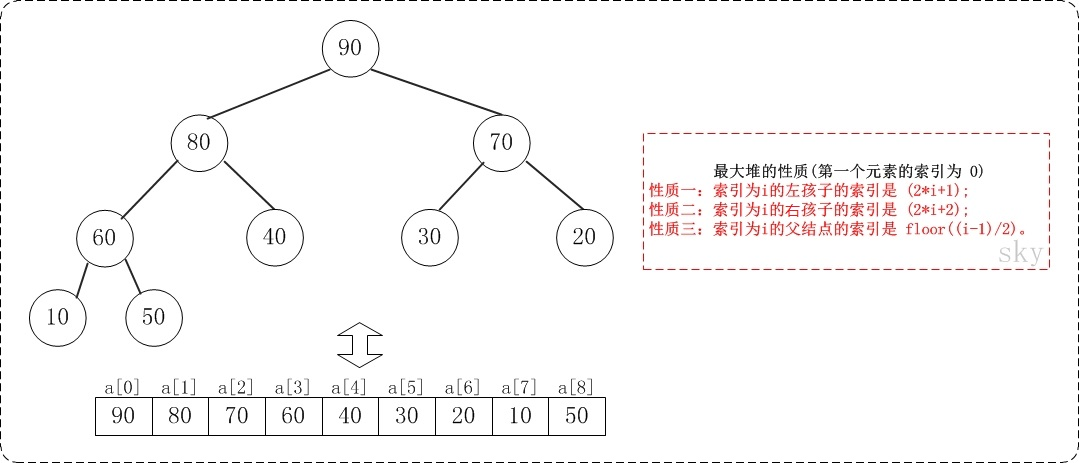• 索引为i的左孩子的索引是 (2*i);
• 索引为i的右孩子的索引是 (2*i+1);
• 索引为i的父结点的索引是 floor(i/2);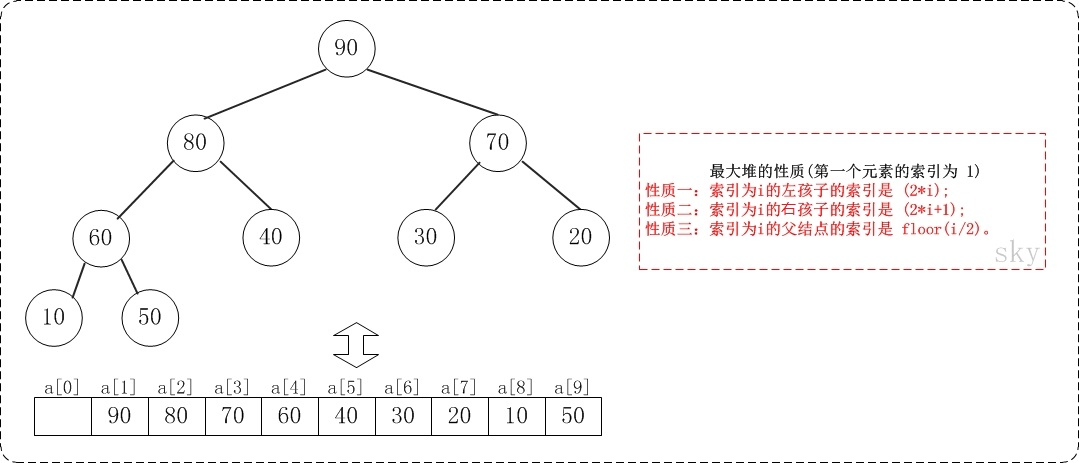### 2.1 基本操作

• MinBinaryHeap()：创建一个空的二叉堆对象
• min_insert(self,data)：将新元素加入到堆中
• remove(self,data)：删除堆中某个元素
• minChild(self, i)：返回子树中最小的索引值
• buildHeap(list)：从一个包含节点的列表里创建新堆

### 2.2 操作的实现

#### 2.2.1 二叉堆结构

class MinBinaryHeap(object):
def __init__(self):
self.heaplist = []
self.currentsize = 0


#### 2.2.2 插入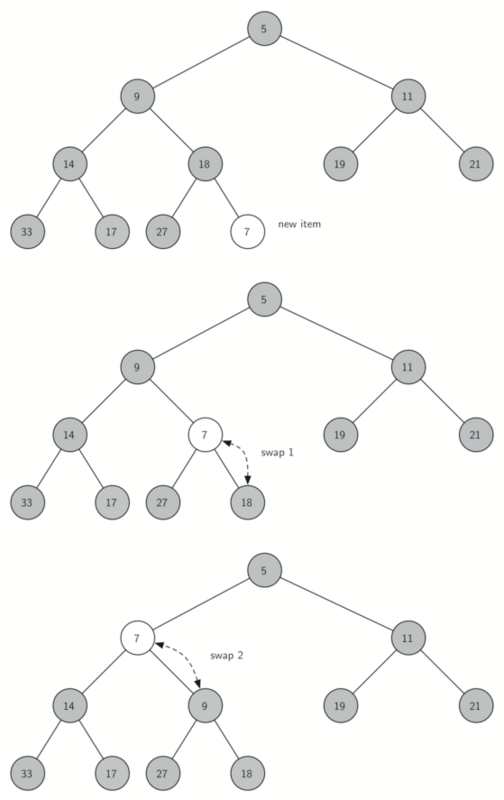# “上浮”方法，它把一个新节点“上浮”到其正确位置来满足堆次序。
def min_percUp(self, n):
# 一直循环到根节点，也就是数组中索引为0的数值，当索引小于0则不在数组中跳出循环
while ((n - 1) // 2) >= 0:
# 比较这个节点和父节点的大小
if self.heaplist[n] < self.heaplist[(n - 1) // 2]:
temp = self.heaplist[(n - 1) // 2]
self.heaplist[(n - 1) // 2] = self.heaplist[n]
self.heaplist[n] = temp
n = (n - 1) // 2  # 将指针移到该节点的父节点，然后继续上浮

def min_insert(self, data):
self.heaplist.append(data)
self.currentsize += 1
self.min_percUp(self.currentsize - 1)


#### 2.2.3 删除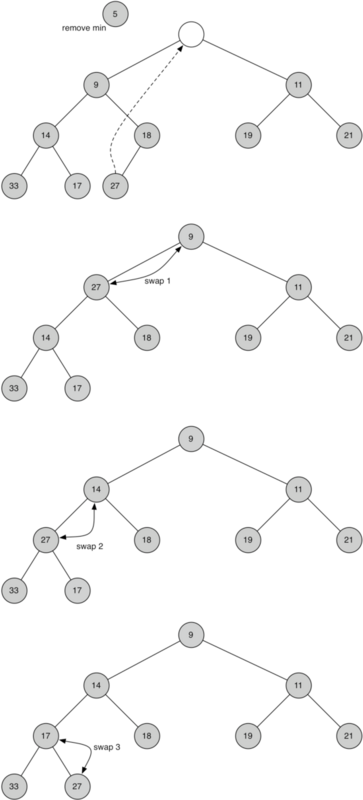# 删除某个节点的数据
def min_delete(self, data):
index = self.heaplist.index(data)  # 先确定删除节点的索引
if index == self.currentsize - 1:  # 当删除节点在列表末尾直接删除
self.heaplist = self.heaplist[:-1]
self.currentsize -= 1
else:
self.heaplist[index] = self.heaplist[-1]  # 将列表末尾数放到删除节点的位置
self.heaplist = self.heaplist[:-1]
self.currentsize -= 1
self.min_percDown(index)

# 返回子树中最小的索引值
def minChild(self, i):
# 如果该节点没有右孩子，那么左孩子就是最小值
if i * 2 + 2 > self.currentsize - 1:
return i * 2 + 1
else:
# 如果存在左右孩子，返回最小的那个
if self.heaplist[i * 2 + 1] < self.heaplist[i * 2 + 2]:
return i * 2 + 1
else:
return i * 2 + 2

# “下沉”操作
def min_percDown(self, n):
# 如果这个节点有左孩子，则进入循环
while n * 2 + 1 <= self.currentsize - 1:
mc = self.minChild(n)  # 确定该节点的最小的孩子的索引值
# 如果该节点值大于孩子节点最小的值，则进行交换
if self.heaplist[n] > self.heaplist[mc]:
temp = self.heaplist[mc]
self.heaplist[mc] = self.heaplist[n]
self.heaplist[n] = temp
n = mc  # 更新当前节点的位置，继续下沉


#### 2.2.4 生成最小堆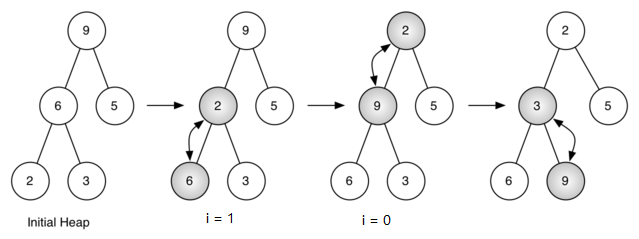def buildminHeap(self, data):
i = len(data) // 2 - 1
self.currentsize = len(data)
self.heaplist = data
while i >= 0:
self.min_percDown(i)
i = i - 1


#### 2.2.5 最小堆的完整实现

class MinBinaryHeap(object):
def __init__(self):
self.heaplist = []
self.currentsize = 0

# “上浮”方法，它把一个新节点“上浮”到其正确位置来满足堆次序。
def min_percUp(self, n):
# 一直循环到根节点，也就是数组中索引为 0的数值，当索引小于 0则不在数组中跳出循环
while ((n - 1) // 2) >= 0:
# 比较这个节点和父节点的大小
if self.heaplist[n] < self.heaplist[(n - 1) // 2]:
temp = self.heaplist[(n - 1) // 2]
self.heaplist[(n - 1) // 2] = self.heaplist[n]
self.heaplist[n] = temp
n = (n - 1) // 2  # 将指针移到该节点的父节点，然后继续上浮

def min_insert(self, data):
self.heaplist.append(data)
self.currentsize += 1
self.min_percUp(self.currentsize - 1)

# 删除某个节点的数据
def min_delete(self, data):
index = self.heaplist.index(data)  # 先确定删除节点的索引
if index == self.currentsize - 1:  # 当删除节点在列表末尾直接删除
self.heaplist = self.heaplist[:-1]
self.currentsize -= 1
else:
self.heaplist[index] = self.heaplist[-1]  # 将列表末尾数放到删除节点的位置
self.heaplist = self.heaplist[:-1]
self.currentsize -= 1
self.min_percDown(index)

# 返回子树中最小的索引值
def minChild(self, i):
# 如果该节点没有右孩子，那么左孩子就是最小值
if i * 2 + 2 > self.currentsize - 1:
return i * 2 + 1
else:
# 如果存在左右孩子，返回最小的那个
if self.heaplist[i * 2 + 1] < self.heaplist[i * 2 + 2]:
return i * 2 + 1
else:
return i * 2 + 2

# “下沉”操作
def min_percDown(self, n):
# 如果这个节点有左孩子，则进入循环
while n * 2 + 1 <= self.currentsize - 1:
mc = self.minChild(n)  # 确定该节点的最小的孩子的索引值
# 如果该节点值大于孩子节点最小的值，则进行交换
if self.heaplist[n] > self.heaplist[mc]:
temp = self.heaplist[mc]
self.heaplist[mc] = self.heaplist[n]
self.heaplist[n] = temp
n = mc  # 更新当前节点的位置，继续下沉

def buildminHeap(self, data):
i = len(data) // 2 - 1
self.currentsize = len(data)
self.heaplist = data
while i >= 0:
self.min_percDown(i)
i = i - 1


#### 2.2.6 最大堆的完整实现

class MaxBinaryHeap(object):
def __init__(self):
self.heaplist = []
self.currentsize = 0

def max_percUp(self, i):
while ((i - 1) // 2) >= 0:
if self.heaplist[i] > self.heaplist[(i - 1) // 2]:
temp = self.heaplist[(i - 1) // 2]
self.heaplist[(i - 1) // 2] = self.heaplist[i]
self.heaplist[i] = temp
i = (i - 1) // 2

def max_insert(self, data):
self.heaplist.append(data)
self.currentsize += 1
self.max_percUp(self.currentsize - 1)

def max_delete(self, data):
index = self.heaplist.index(data)
if index == self.currentsize - 1:
self.heaplist = self.heaplist[:-1]
self.currentsize -= 1
else:
self.heaplist[index] = self.heaplist[-1]
self.heaplist = self.heaplist[:-1]
self.currentsize -= 1
self.max_percDown(index)

def maxChild(self, i):
if i * 2 + 2 > self.currentsize - 1:
return i * 2 + 1
else:
if self.heaplist[i * 2 + 1] > self.heaplist[i * 2 + 2]:
return i * 2 + 1
else:
return i * 2 + 2

def max_percDown(self, i):
while i * 2 + 1 <= self.currentsize - 1:
mc = self.maxChild(i)
if self.heaplist[i] < self.heaplist[mc]:
temp = self.heaplist[mc]
self.heaplist[mc] = self.heaplist[i]
self.heaplist[i] = temp
i = mc

def buildmaxHeap(self, data):
i = len(data) // 2 - 1
self.heaplist = data
self.currentsize = len(data)
while i >= 0:
self.max_percDown(i)
i = i - 1